Scattering and tunnelling

This free course is available to start right now. Review the full course description and key learning outcomes and create an account and enrol if you want a free statement of participation.

Free course

# 4.3 Stationary states and barrier penetration

The example of tunnelling we have just been examining can be regarded as a special case of scattering; it just happens to have E0 < V0. As long as we keep this energy range in mind, we can apply the same stationary-state methods to the study of tunnelling that we used earlier when studying scattering.

As before, we shall start by considering the finite square step, whose potential energy function was defined in Equations 7.6 and 7.7. This is shown for the case E0 < V0 in Figure 19. The potential energy function divides the x-axis into two regions: Region 1 (x ≤ 0) which contains the incident and reflected beams, and Region 2 (x > 0) which contains what is effectively an infinitely wide barrier. There is no possibility of tunnelling through the barrier in this case since there is no classically allowed region on the far side, but the finite square step nonetheless constitutes a valuable limiting case, as you will see.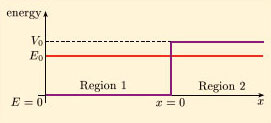Figure 19 A finite square step potential energy function. Also shown is the energy E0 of an incident particle for the case E0 < V0, where V0 is the height of the step

Proceeding as before, we seek stationary-state solutions of the Schrödinger equation of the form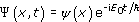, where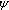(x) is a solution of the corresponding time-independent Schrödinger equation. In this case we might try to use exactly the same solution as in Section 3.3 but doing so would complicate the analysis since we would find that in the region x > 0 the wave number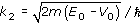would be imaginary. In view of this, it is better to recognise that E0 < V0 generally implies a combination of exponentially growing and exponentially decaying terms in the region x > 0, and write the solutions as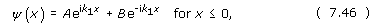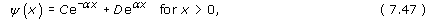where, A, B, C and D are arbitrary complex constants, while k1 and α are real quantities given by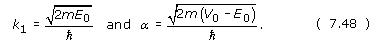We require D to be zero on physical grounds, to avoid having any part of the solution that grows exponentially as x approaches infinity. To determine the values of B and C relative to that of A we impose the usual requirement (for a finite potential energy function) that both(x), and its derivative d/dx, must be continuous everywhere. Applying these conditions at x = 0 we find: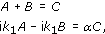from which it follows that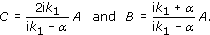The reflection coefficient is given by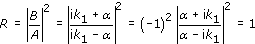(Note: For any complex number, z, )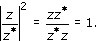So, if particles of energy E0 < V0 encounter a finite square step of height V0, reflection is certain. There is no transmission and no possibility of particles becoming lodged inside the step; everything must eventually be reflected. Note however that(x) is not zero inside the step (see Figure 20). Rather, it decreases exponentially over a length scale determined by the quantity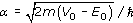, which is usually called the attenuation coefficient. This is an example of the phenomenon of barrier penetration. It is not the same as tunnelling since there is no transmitted beam, but it is what makes tunnelling possible, and the occurrence of exponentially decaying solutions in a classically forbidden region suggests why tunnelling probabilities decline rapidly as barrier width increases.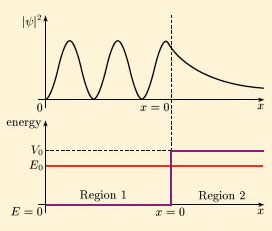Figure 20 The quantity |ψ(x)|2 plotted against x for a finite square step in the case E0 < V0. There is a finite probability that the particle will penetrate the step, even though there is no possibility of tunnelling through it

## Exercise 10

Show that the stationary-state probability density |(x)|2 in Region 1 of Figure 20 is a periodic function of x with minima separated by/k1.

### Answer

From Equation 7.46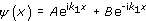and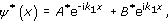so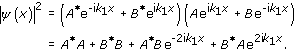This is a periodic function that runs through the same range of values each time x increases by 2/2k1, so its minima are separated by/k1.

## Exercise 11

Show that the probability current in Region 2 of Figure 20 is zero.

### Answer

Using Equation 7.47, with D = 0 and α real, and recalling the definition of probability current given in Equation 7.28, we have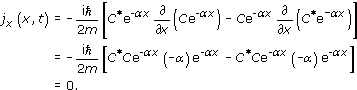SM358_1

### Take your learning further

Making the decision to study can be a big step, which is why you'll want a trusted University. The Open University has 50 years’ experience delivering flexible learning and 170,000 students are studying with us right now. Take a look at all Open University courses.

If you are new to university level study, find out more about the types of qualifications we offer, including our entry level Access courses and Certificates.

Not ready for University study then browse over 900 free courses on OpenLearn and sign up to our newsletter to hear about new free courses as they are released.

Every year, thousands of students decide to study with The Open University. With over 120 qualifications, we’ve got the right course for you.

Request an Open University prospectus